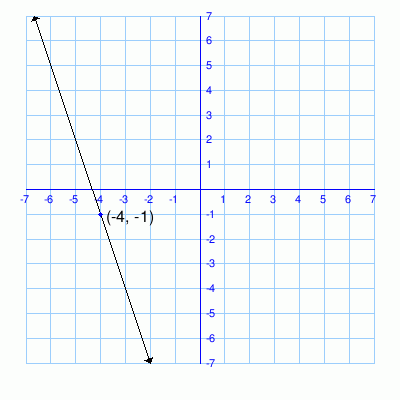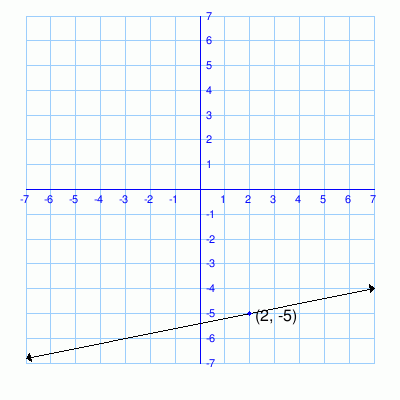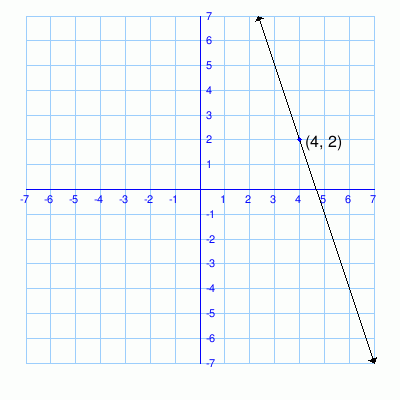# Math Practice Topic: Graphs to Linear Equations 2

 Description: Determine the linear equation for a graphed line in point slope form: y-y1=m(x-x1). Point slope form is the easiest format for taking a slope and any intersection point and making an equation. Adaptive Learning Progression: Number of problems increases. Start using MathScore for free

## Sample Levels (out of 4)

Write the equation in point slope format, y - y1 = m(x - x1), then convert into slope intercept form, y = mx + b.

 1Point Slope Equation: Slope Intercept Equation:

Write the equation in point slope format, y - y1 = m(x - x1), then convert into slope intercept form, y = mx + b.

 1Point Slope Equation: Slope Intercept Equation: 2Point Slope Equation: Slope Intercept Equation: 3Point Slope Equation: Slope Intercept Equation: